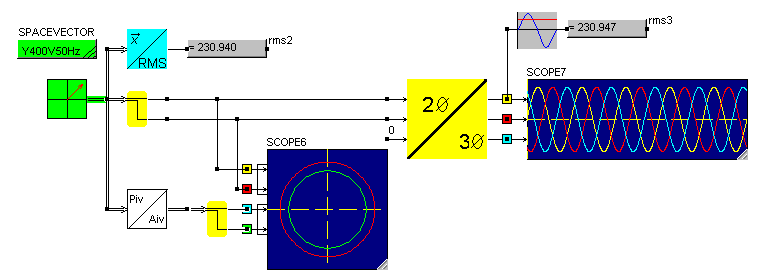• Introduction
• What is in this manual
• What is Caspoc
• User interface
• Introduction
• Starting
• Simulation
• Editing
• Viewing and printing
• Getting Started
• Basic editing
• Simulation in the time domain
• Basic User Interface Topics
• Editing
• Simulation
• Viewing
• Library
• Reports
• Project management
• Circuit and Block Diagram Components
• Introduction
• Cscript and user defined functions
• Component parameters
• Modeling Topics
• Introduction
• Power Electronics
• Semiconductors
• Electrical Machines
• Electrical drives
• Power Systems
• Mechanical Systems
• Thermal Systems
• Magnetic Circuits
• Green Energy
• Coupling to FEM
• Experimenter
• Analog hardware description language
• Embedded C code Export
• Coupling to Spice
• Small Signal Analysis
• Matlab coupling
• Tips and tricks
• Appendices

## Space vector.

The models in the Advanced Electrical Drives library are space vector models. This means that each component and connection is build on the basis of a vector, or better defined a space vector.
A space vector can be defined in a stator reference frame, a two phase signal after a 3/2 Clark transformation, but also in the rotating reference frame commonly referred to as dq .

Power Invariant
The space vector is defined as being power invariant. This means that the product of space vectors for voltage and current gives the power in Watt.

Compared to most other 2 phase systems that are mostly amplitude variant, the amplitude of the phasors are not equal to the amplitude of the original signals.

In a Power Invariant system the amplitude of the phasor equals the RMS value of the line voltage. For example, a three phase Y400V50Hz system has a phasor amplitude of 400[V].
The RMS of one phase equals 231[V] , which is calculated using the RMS component in the Sensor/Vector section.The numerical value of the node itself gives the length of the space vector. In a Y400V50Hz power invariant system, the length of the space vector is 400.